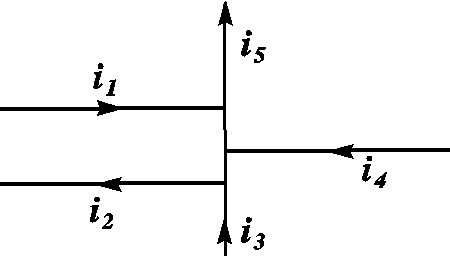# Kirchoff's Junction Rule Help

## Homework StatementCalculate the current i5 in the configuration. Use the following data: i1=8.65 A, i2=5 A, i3=7.85 A, and i4=8.5 A.

## Homework Equations

Kirchoff's junction rule states that the sum of the currents meeting at a junction has to equal zero.

## The Attempt at a Solution

According to the junction rule, i1+i2+i4=i3+i5. When I plugged in the numbers, I got i5=14.3 A but my homework says that is wrong. Does the direction of the arrows affect the outcome or am I overthinking it at this point?

berkeman
Mentor

## Homework StatementCalculate the current i5 in the configuration. Use the following data: i1=8.65 A, i2=5 A, i3=7.85 A, and i4=8.5 A.

## Homework Equations

Kirchoff's junction rule states that the sum of the currents meeting at a junction has to equal zero.

## The Attempt at a Solution

According to the junction rule, i1+i2+i4=i3+i5. When I plugged in the numbers, I got i5=14.3 A but my homework says that is wrong. Does the direction of the arrows affect the outcome or am I overthinking it at this point?

It is better to either add up the currents leaving a node, or add up the currents entering a node. I personally like to add the currents leaving a node and set that sum equal to zero. So yes, the arrow directions definitely do make a difference. Since I like to add up the currents leaving a node, I would use a + sign on all of the currents in the figure that are leaving the node, and a - sign on the currents that are entering the node. The sum of thoses currents (with either a + or - sign in front of each) has to equal zero.

$$\Sigma I_n = 0$$

So for my particular example:
Entering the node: i1, i4, and i3? i3 and i5 confuse me. When I add up the total, I get -i1+i2-i3-i4=-20. So i5 would have to be 20 for the total to equal zero. I feel like I am still missing something here.

berkeman
Mentor
So for my particular example:
Entering the node: i1, i4, and i3? i3 and i5 confuse me. When I add up the total, I get -i1+i2-i3-i4=-20. So i5 would have to be 20 for the total to equal zero. I feel like I am still missing something here.

That is all correct, IMO. So you write the equation like this:

-i1+i2-i3-i4+i5=0

And solve for i5. That gives you the answer you mention. Is it correct?

The problem with the diagram is that it is hard to see a node.

A node is basically a point in space where the different circuit elements meet.

Notice how i3 and i5 are heading to the same direction but they are different in magnitude.

This is when you know there is a node(s) between.

Thanks so much guys! 20 was correct and I understand why. :)